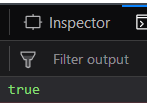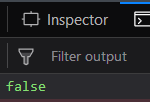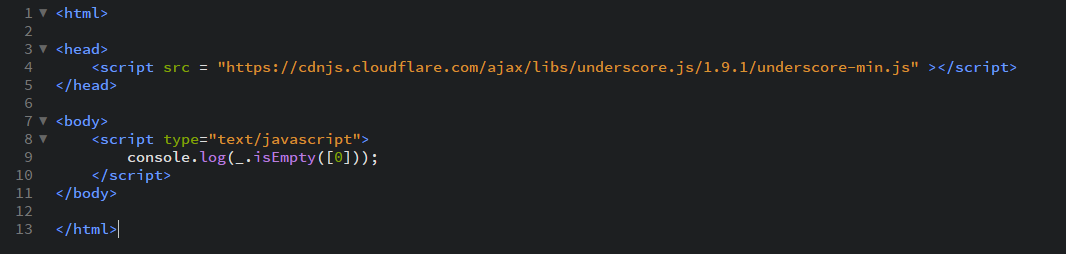Open in App
Not now

# Underscore.js _.isEmpty() Function

• Last Updated : 20 Dec, 2021

_.isEmpty() function:

• It is used to check whether a list, array, string, object etc is empty or not.
• It first finds out the length of the passed argument and then decides.
• If length is zero, then the output is true otherwise false.

Syntax:

`_.isEmpty(object)`

Parameters:
It takes only one argument which is the object.
Return value:
It returns true if the argument passed is empty, i.e., does not have any elements in it. Otherwise it returns false.
Examples:

• Passing an empty element to the _.isEmpty() function:
The _.isEmpty() function takes the element from the list one by one and starts counting the length of the array. Each time it encounters an element, it increments length by one. Then, when the array finishes, it checks if the array’s length is zero ( it returns true) or greater than zero (then, it returns false). Here, we have an empty array so the output will be true.

## html

 ```<``html``>`` ` `<``head``>``    ``<``script` `src =``    ``"https://cdnjs.cloudflare.com/ajax/libs/underscore.js/1.9.1/underscore-min.js">``     `````` ` `<``body``>``    ``<``script` `type="text/javascript">``        ``console.log(_.isEmpty([]));``    `````` ` ``

Output:• Passing an array with 6 elements to the _.isEmpty() function:
The procedure for the check function will be same as in the above example. Here, we have 6 elements in the array which implies that at the finish of the array, it’s length will be 6. so, the length is not equal to 0 and hence the answer will be false.

## html

 ```<``html``>`` ` `<``head``>``    ``<``script` `src =``    ``"https://cdnjs.cloudflare.com/ajax/libs/underscore.js/1.9.1/underscore-min.js">``    `````` ` `<``body``>``    ``<``script` `type="text/javascript">``        ``console.log(_.isEmpty([1, 2, 3, 4, 5, 6]));``    `````` ` ``

Output:• Passing a list of characters to _.isEmpty() function:
The _.isEmpty() function will work the same as in the examples above. It implies that it does not distinguish between whether the array has numbers, characters or is empty. It will work the same on all the array and find out their length. In this example we have an array of length 4. Hence, the output will be false.

## html

 `<``html``>`` ` `<``head``>``    ``<``script` `src =``    ``"https://cdnjs.cloudflare.com/ajax/libs/underscore.js/1.9.1/underscore-min.js">``     `````` ` `<``body``>``    ``<``script` `type="text/javascript">``        ``console.log(_.isEmpty(['HTML', 'CSS', 'JS', 'AJAX']));``    `````` ` ``

Output:• Passing an element zero to the _.isEmpty() function:
Do not get confused with the empty array and an array containing zero as an element. Since the elements is zero, so you must be thinking that the array is empty. But the array contains an element and since the _isEmpty() calculates the length, therefore the length of the below array will be one which is greater than zero. And hence the output will be false.

## html

 `` `<``html``>`` ` `<``head``>``    ``<``script` `src =``    ``"https://cdnjs.cloudflare.com/ajax/libs/underscore.js/1.9.1/underscore-min.js">``     `````` ` `<``body``>``    ``<``script` `type="text/javascript">``        ``console.log(_.isEmpty());``    `````` ` ``

Output:NOTE:
These commands will not work in Google console or in firefox as for these additional files need to be added which they didn’t have added.
 ```<``script` `type="text/javascript" src =``"https://cdnjs.cloudflare.com/ajax/libs/underscore.js/1.9.1/underscore-min.js">```